# Basic Arithmetic : Increase

## Example Questions

### Example Question #1 : Finding Percent Change

In 2012, a university received 150,249 applicants. In 2013, the same university received 190,201 applicants. By what percent did the total number of applicants increase from 2012 to 2013?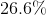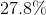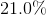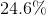Explanation:

To find the percent change, use the following formula: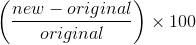Our new number of applicants is 190,201, while the original number of applicants is 150,249.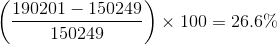### Example Question #1 : Finding Percent Change

In January, Widget Company made,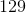widgets. In February, the same company made,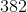widgets. By what percentage did the amount of widgets made increase?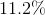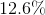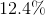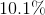Explanation:

To find the percent increase, use the following formula: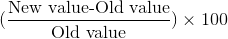In the case of Widget Company,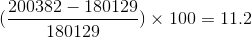Therefore, the percent increase is### Example Question #2 : Finding Percent Change

Derek ran his first mile inminutes and his second mile inminutes.

What is the percent increase between the time it took Derek to run the first and second mile?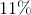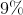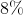Explanation:

The correct answer is a percent change (increase) of.

This can be calculated using the following equation: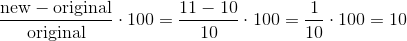Therefore, the answer is a percent increase of.

### Example Question #1 : Finding Percent Change

In May of the past year, Harry's company made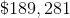in profit, and in June of that same year, his company made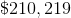in profit. By what percent did his profit increase from May to June?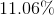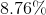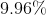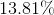Explanation:

To find percent change, use the following formula: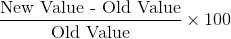Our new value is the profit in June, $210,219. Our old value is the profit in May,$189,281.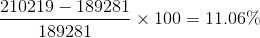### All Basic Arithmetic Resources